# Volume of a Sphere

The Volume of a Sphere

A sphere is a set of points in space that are a given distance r from the center.

The volume of Sphere is the amount of space it occupies.  Volume is measured in cubic units (in3,ft3,cm3,m3 etc).

The volume V of a sphere is four-thirds times pi times the radius cubed.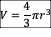The volume of a hemisphere is one-half of the volume of the related sphere.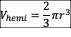Example 1:

Find the volume of the sphere. Round to the nearest cubic meter.
Solution

The formula for the volume of a sphere is…From the figure, the radius of the sphere is 8 m.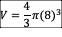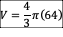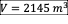Example 2:

A sphere has a volume of 500 cm3. Calculate the radius of the sphere. Round your answer to the nearest whole number.
Solution

The formula for the volume of a sphere is…Substitute V = 500…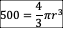Re-arrange for r…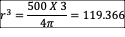The opposite of the cubic power is the cubed root…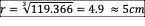Need more help with Geometry?